IMO Shortlist 2010 problem N6

Kvaliteta:
Avg: 0.0
Težina:
Avg: 8.0
The rows and columns of a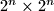$2^n \times 2^n$ table are numbered from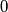$0$ to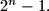$2^{n}-1.$ The cells of the table have been coloured with the following property being satisfied: for each$0 \leq i,j \leq 2^n - 1,$ the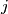$j$-th cell in the$i$-th row and the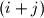$(i+j)$-th cell in the$j$-th row have the same colour. (The indices of the cells in a row are considered modulo$2^n$.) Prove that the maximal possible number of colours is$2^n$.

Proposed by Hossein Dabirian, Sepehr Ghazi-nezami, Iran
Source: Međunarodna matematička olimpijada, shortlist 2010